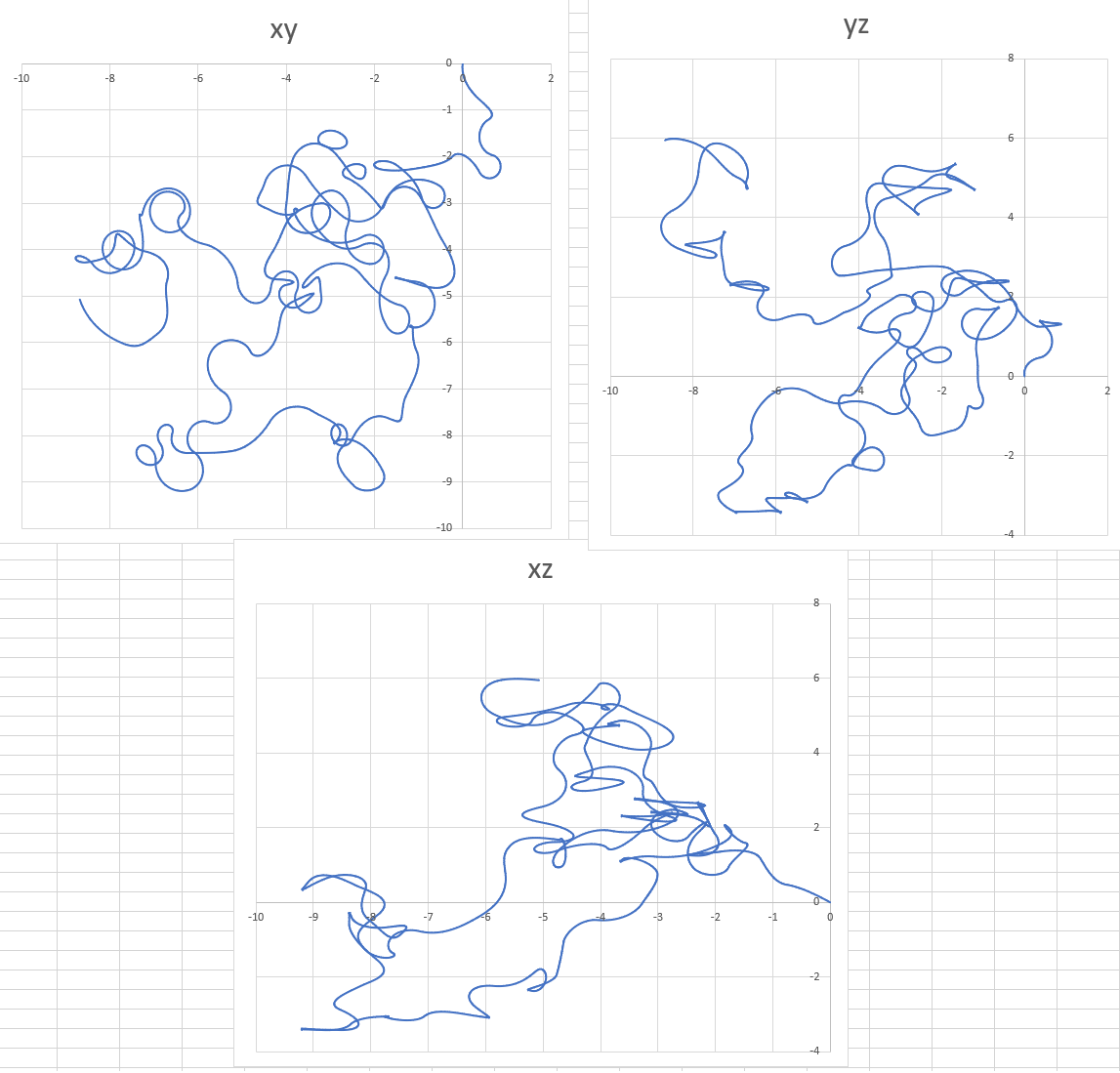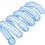# 3D Random Curves from Physics

Here is some code for generating random 3D curves based on physics. The code does the following:

1) Initialize a particle with mass, charge, position, and velocity in 3D space
2) Have a magnetic flux density $\vec{B}$ which randomly changes magnitude and direction once per second
3) Calculate the force on the particle and simulate the trajectory

Since the magnetic field does no work, the speed of the particle remains constant. Since the curve is 3D, each run of the simulation results in three curves (projections on the xy, yz, and xz planes). One such set of curves is shown below (click to enlarge). Each run produces a different curve, since the B field changes randomly. The program spits out spatial coordinates which can be plotted in Excel.1 2 3 4 5 6 7 8 9 10 11 12 13 14 15 16 17 18 19 20 21 22 23 24 25 26 27 28 29 30 31 32 33 34 35 36 37 38 39 40 41 42 43 44 45 46 47 48 49 50 51 52 53 54 55 56 57 58 59 60 61 62 63 64 65 66 67 68 69 70 71 72 73 74 75 76 77 78 79 80 81 82 83 84 85 import math import random # Constants B = 3.0 v = 1.0 m = 1.0 q = 1.0 dt = 10.0**(-3.0) ######################################## # Initialize Simulation t = 0.0 count = 0 thetav = 2.0*math.pi*random.random() phiv = math.pi*random.random() thetaB = 2.0*math.pi*random.random() phiB = math.pi*random.random() x = 0.0 y = 0.0 z = 0.0 vx = v*math.cos(thetav)*math.sin(phiv) vy = v*math.sin(thetav)*math.sin(phiv) vz = v*math.cos(phiv) Bx = B*math.cos(thetaB)*math.sin(phiB) By = B*math.sin(thetaB)*math.sin(phiB) Bz = B*math.cos(phiB) Fx = q*(vy*Bz - vz*By) Fy = -q*(vx*Bz - vz*Bx) Fz = q*(vx*By - vy*Bx) ax = Fx/m ay = Fy/m az = Fz/m ######################################## # Run simulation and plot points print "t x y z" while t <= 100.0: x = x + vx*dt y = y + vy*dt z = z + vz*dt vx = vx + ax*dt vy = vy + ay*dt vz = vz + az*dt Fx = q*(vy*Bz - vz*By) Fy = -q*(vx*Bz - vz*Bx) Fz = q*(vx*By - vy*Bx) ax = Fx/m ay = Fy/m az = Fz/m if count % 1000 == 0: B = 1.0 + 3.0*random.random() thetaB = 2.0*math.pi*random.random() phiB = math.pi*random.random() Bx = B*math.cos(thetaB)*math.sin(phiB) By = B*math.sin(thetaB)*math.sin(phiB) Bz = B*math.cos(phiB) if count % 10 == 0: print t,x,y,z t = t + dt count = count + 1Note by Steven Chase
1 year, 1 month ago

This discussion board is a place to discuss our Daily Challenges and the math and science related to those challenges. Explanations are more than just a solution — they should explain the steps and thinking strategies that you used to obtain the solution. Comments should further the discussion of math and science.

When posting on Brilliant:

• Use the emojis to react to an explanation, whether you're congratulating a job well done , or just really confused .
• Ask specific questions about the challenge or the steps in somebody's explanation. Well-posed questions can add a lot to the discussion, but posting "I don't understand!" doesn't help anyone.
• Try to contribute something new to the discussion, whether it is an extension, generalization or other idea related to the challenge.

MarkdownAppears as
*italics* or _italics_ italics
**bold** or __bold__ bold
- bulleted- list
• bulleted
• list
1. numbered2. list
1. numbered
2. list
Note: you must add a full line of space before and after lists for them to show up correctly
paragraph 1paragraph 2

paragraph 1

paragraph 2

[example link](https://brilliant.org)example link
> This is a quote
This is a quote
    # I indented these lines
# 4 spaces, and now they show
# up as a code block.

print "hello world"
# I indented these lines
# 4 spaces, and now they show
# up as a code block.

print "hello world"
MathAppears as
Remember to wrap math in $$ ... $$ or $ ... $ to ensure proper formatting.
2 \times 3 $2 \times 3$
2^{34} $2^{34}$
a_{i-1} $a_{i-1}$
\frac{2}{3} $\frac{2}{3}$
\sqrt{2} $\sqrt{2}$
\sum_{i=1}^3 $\sum_{i=1}^3$
\sin \theta $\sin \theta$
\boxed{123} $\boxed{123}$

Sort by:

@Steven Chase yeah it seems me interesting.
Today are you posting any new problem. Please reply otherwise I have to keep checking.
If you are posting can you post a LRC circuit second order problem?

- 1 year, 1 month ago

Hey bro, you should pursue physics or engineering when you get older. Use your talent! I'm probably going to be a theoretical physicist when I leave high school😁

- 1 year, 1 month ago

Hey, good stuff!

- 1 year, 1 month ago

Thanks. Each new curve has never been seen before, and will never be seen again

- 1 year, 1 month ago

Random indeed!

- 1 year, 1 month ago

Nice note. I remember you sharing insights about generating random curves, a few months back.

- 1 year, 1 month ago

@Karan Chatrath Sir please post the analytical solution of this Problem.

- 1 year, 1 month ago

The equations of motion cannot be solved analytically from my point of view. They are nonlinear and coupled. However, you could try deriving the equations of motion. That would be a nice exercise.

- 1 year, 1 month ago

@Karan Chatrath I have some question
1) Which version of python you use?
2) Did you have taken the membership of MATLAB, I have seen it's very expensit. Can I get the pirated version from anywhere??

- 1 year, 1 month ago

Engineering colleges and universities all over the world give students access to MATLAB and that is the way I use that software too. Obtaining a pirated version of the software is highly unethical and I will not endorse that.

Python, on the other hand, is freely available. I do not use it, but from my limited understanding, the version matters less, especially so if you want to perform simple numerical analyses.

- 1 year, 1 month ago

@Karan Chatrath so will you give me that access to me??? Please I just want to know from where can I get???

- 1 year, 1 month ago

I do not think it is possible for a school-going student to obtain the software.

- 1 year, 1 month ago

@Karan Chatrath Ok sir.
But why?

- 1 year, 1 month ago

It is because schools do not usually have a MATLAB licence. You may check with yours, but I think it is unlikely. You rightly said that an individual licence is very expensive so the only way to get it is when you start your Bachelor studies if that is what you plan to do. Engineering colleges and technical institutions do have licences which their students can access.

- 1 year, 1 month ago

Yes, those were 2D curves. I realized that a changing magnetic field was the perfect thing to generate 3D random curves

- 1 year, 1 month ago